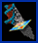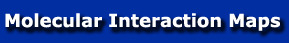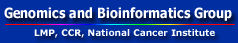Kohn Interaction Maps MIM home Kohn Interaction Maps Map A: Cyclins & E2F Map B: p53 & DNA repair Symbol Definition Table Annotation List References Molecular Species Index Abbreviations RNfb program files INDEX OF MONOMOLECULAR SPECIES Index of monomolecular species (Bold letters refer to maps A or B of Figure 6A or B, respectively. The number-letter combination that follows the bold letter is the location on the grid. Italics refer to locations where the interactions of the molecular species are included in abbreviated form only.)   14-3-3. A2K, B11E Abl. A7A, B5E AP2. A10C APC. A3I ATM. B5D Bax. B10H BRCA1. B10F Caspase 3. B7K CBF3. A1G, B3B Cdc25A. A4C Cdc25C. A4H Cdc2, see Cdk1 Cdk1. A4I Cdk2. A4G, B1F, B9B, B10D Cdk4/6. A4D, B1E Cdk7. A2C, B1C C/EBP. A8E Chk1. A5H CK1d/k. B7B CK2. B7C Cks1. A5I Crk. B5E CSB. B1C C-TAK1. A5H Cyclin A. A3H, B1F, B2K, B9B Cyclin B. A3H Cyclin D. A3D, B1E Cyclin E. A3F, B1F, B10D Cyclin H. A1C, B1C DMP1. A3C, B6G DNA-PK. B3E DP1/2. A9G, B6F, B6I , B10D DPase a. B4B DPase b. B4I DPase d. B4A dsDNA ends. B3D E2F1,2,3. A7G, B6I, B10D E2F4. A7H E2F5. A7H E2F6. A7H E-cadherin. A10D ERCC1. A9D, B3I FEN-1. B5A Fos. A8C, B11I Gadd45. A3D, B5B HBP1. A8F HDAC1. A10H, B11C Histones A10H, B11B HMG. B11C HR23B. B4I JNK. B7B Jun. A8C Karp-1, B3F Ku70. B3F Ku80. B3F Ligase I. B5B Ligase III. B4I MAPK. B7B MAT1, see p36MAT1 Max. A10C Mdm2. A1I. B7I Myc. A9C Myt1. A4J p107. A7E p130. A7F p14ARF, see p19ARF p16. A4B, B1E p19ARF. A6I. B8G p21Cip1. A3E, B1F, B11H p27. A3E p300. A10H, B10B p36MAT1. B1C p53. A1H. B8E p57. A3F p68. A1F, B4B PARP. B5J Paxillin. B5E pCAF. A10G, B10B PCNA. A1E, B5B PKC. B7C PKCa/b. B5H Plk1. A3J Pol a. A1F pRb. A7D, B5F, B6J, B10E Primase. A1F, B4B Rad51. B3K Rad52. B3J Raf1. A6A Ras. A5A Rb, see pRb Replication fork. B4A RF-C. B5A Rb, see pRb RHA. B10D RPA. A1E RPA1. B1H RPA2. B2J RPA3. B1J RPase II. B1A Skp1. A1G, B3B Skp2. A3G, B2B SL1. A1B Sp1. A7J ssb. B5H ssDNA. B1G, B9G TAFII250. B1B TBP. B1B TFIIH. B1B U-glycosylase, B2I Wee1. A3J XPA. B3I XPB. B1C XPC. B4H XPD. B1C XPF. B4H XPG. B3H XRCC1. B4J